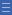# Activity: Reach 100

Activity: Reach 100

Your challenge is to place four different digits from 1 to 9 in a 2 x 2 grid that give four two-digit numbers which add to a total of 100.

## Find the activity on NRICH

Go to http://nrich.maths.org/1130 (link will open in a new window)

## More questions

What digits should you place in each square to make the largest sum possible? Is there more than one way to do this? What about the smallest sum?

Are all totals between the smallest and the largest ones possible? If not, which ones aren’t?

If you place the digits 1 to 9 in a 3 x 3 grid what is the smallest possible sum of the six 3-digit numbers formed by the rows and columns? Can you make the sum 2000?

## Shine a light on the Proficiencies

This problem requires strategic thinking to decide where to place the digits to make the smallest and largest sums. The explanation of where to place the digits can be done formally or informally, and is an ideal opportunity to use the concept of place value to reason mathematically.

The problem lends itself to an algebraic formulation in the secondary years.

Yes

Yes

Name Class Section PriorityDownload Reach 100 (blank worksheet) File 1